How to Learn in 24 Hours?The Rapid Learning Movie

 Need Help? M-F: 9am-5pm(PST): Toll-Free: (877) RAPID-10 US Direct: (714) 692-2900 Int'l: 001-714-692-2900 24/7 Online Technical Support: The Rapid Support Center Secure Online Order:Need Proof? Testimonials by Our Users

 Rapid Learning Courses: MCAT in 24 Hours (2015-16) USMLE in 24 Hours (Boards) Chemistry in 24 Hours Biology in 24 Hours Physics in 24 Hours Mathematics in 24 Hours Psychology in 24 Hours SAT in 24 Hours ACT in 24 Hours AP in 24 Hours CLEP in 24 Hours DAT in 24 Hours (Dental) OAT in 24 Hours (Optometry) PCAT in 24 Hours (Pharmacy) Nursing Entrance Exams Certification in 24 Hours eBook - Survival Kits Audiobooks (MP3)

 Tell-A-Friend: Have friends taking science and math courses too? Tell them about our rapid learning system.

 FAIL (the browser should render some flash content, not this).Home »  Chemistry »  College Chemistry

Electrochemistry

 Topic Review on "Title": Electrochemistry studies how chemical and electrical energy are converted. Oxidation numbers & Redox reactions Oxidation numbers are the charge on an atom if the electrons involved in the bond are assigned to the more electronegative atom in the bond.  When oxidation numbers change during a chemical reaction, it is a redox reaction. Oxidation number method of balancing The oxidation number method is used for balancing simple redox reactions that cannot be easily balanced by the inspection method.  It includes: Determine the oxidation numbers of each atom.  Determine the net change in charge.  Use the net change to determine the ratio of atoms that would cancel out the net charge change. Use the ratio as coefficients in the simplest compounds containing those elements. Finish balancing by the inspection method. Half-reaction method of balancing The half-reaction method is for the most difficult redox reactions: Use oxidation numbers to determine what’s oxidized and what’s reduced. Write two half-reactions, one for reduction and one for oxidation Balance all elements except H and O using inspection method. For an acid redox reaction: Balance the O’s by adding H2O to the side needing more O.  For a base redox reaction: Balance O by adding twice as many OH- to the side needing more O. For a acid redox reaction: Balance the H’s by adding H+ to the side needing more H’s.  For a base redox reaction:  Balance H’s by adding H2O to the side needing more H’s. Determine the charge of each side of each reaction.  Balance the charges by adding electrons to the side with the more positive charge for each reaction. Multiply the half-reactions by factors that will allow the electrons to cancel out. Add the two half-reactions back together. Voltaic cells A voltaic cell separates the reduction and oxidation reaction and forces the electrons to flow over a wire (producing electricity) from the oxidation reaction (at the anode) to the reduction reaction (at the cathode).  The cell consists of the two separate half reaction, metal electrodes and a wire for conducting the electrons, and a salt bridge for balancing the charge build-up to extend the time the cell will operate.  Line notation is a short-hand way of describing a cell: Anode written first Reactants written 1st on each side Anode & Cathode separated with ║ Different states of matter within same side separated with │ Same states of matter within same side separated with a comma Cell potentials The cell potential (or electromotive force) of a voltaic cell is due to the potential energy difference of the electrons before the transfer and after the transfer.  A standard reduction potential is the potential that would be produced between a given half-reaction and hydrogen (hydrogen’s standard reduction potential has been defined as 0).  The standard reduction potentials can be used to calculate the cell potential: EMF = cathode – anode.  Positive EMF values indicate a spontaneous process. Electrolytic cells An electrolytic cell is the opposite of a voltaic cell.  An electrolytic cell converts electrical energy into chemical energy by forcing a reaction to proceed in the non-spontaneous direction by putting electricity in.  The voltage need to force the reaction in the opposite direction is at least that produced by the spontaneous process. Electrochemistry and free energy The free energy of a system can be defined as the amount of work that can be done by the system.  The flow of electrons can do work.  Therefore, the free energy of the system can be defined as: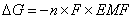DG = free energy (in J); n = # of moles of electrons transferred; F = 1 Faraday;  EMF = cell potential. Electrochemistry and equilibrium When a cell reaches equilibrium, the cell stops reacting.  The Nernst equation relates EMF to equilibrium: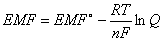EMF = cell potential at current conditions; EMF° = cell potential at standard state (1 atm & 25°C); R = 8.31 J/mole´K; T = temperature (in Kelvin); n = moles electrons transferred; F = 1 Faraday; Q = reaction quotient A cell stops when it reaches equilibrium.  At equilibrium, EMF = 0 and Q = K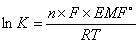where K = equilibrium constant

Rapid Study Kit for "Title":
 Flash Movie Flash Game Flash Card Core Concept Tutorial Problem Solving Drill Review Cheat Sheet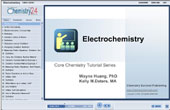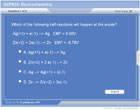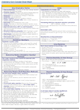"Title" Tutorial Summary : Electrochemistry is the study of the inter-change between chemical and electrical energy.  This tutorial begins with a review of oxidation states, redox reactions, and balancing redox reactions from earlier tutorials.  Voltaic cells, line notation standard cell potential and electromotive force (cell potential) are explained, along with electrolytic cells.  Electrochemistry is then related to free energy and equilibrium.

 Tutorial Features: Specific Tutorial Features: Review of redox content from earlier tutorials Particle animation of voltaic cell and explanation of components of cell.  Series Features: Concept map showing inter-connections of new concepts in this tutorial and those previously introduced. Definition slides introduce terms as they are needed. Visual representation of concepts Animated examples—worked out step by step A concise summary is given at the conclusion of the tutorial.

 "Title" Topic List: Review Oxidation states Redox reaction Balancing redox reactions Voltaic cells Composition Cell potentials Standard reduction potential Voltaic cell potential Electrolytic cells Electrochemistry and free energy Electrochemistry and equilibrium

See all 24 lessons in college chemistry, including concept tutorials, problem drills and cheat sheets:
Teach Yourself College Chemistry Visually in 24 Hours